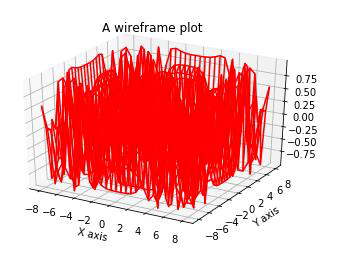# Explain how Matplotlib can be used to create a wireframe plot in Python?

Matplotlib is a popular Python package that is used for data visualization. Visualizing data is an important steps since it helps understand what is going on in the data without actually looking at the numbers and performing complicated computations. It helps in communicating the quantitative insights to the audience effectively.

Matplotlib is used to create 2 dimensional plots with the data. It comes with an object oriented API that helps in embedding the plots in Python applications. Matplotlib can be used with IPython shells, Jupyter notebook, Spyder IDE and so on. It is written in Python. It is created using Numpy, which is the Numerical Python package in Python.

Python can be installed on Windows using the below command −

pip install matplotlib

The dependencies of Matplotlib are −

Python ( greater than or equal to version 3.4)
NumPy
Setuptools
Pyparsing
Libpng
Pytz
Free type
Six
Cycler
Dateutil

Let us understand how Matplotlib can be used to create a wireframe plot −

## Example

from mpl_toolkits import mplot3d
import numpy as np
import matplotlib.pyplot as plt
def my_fun(x, y):
return np.sin(np.sqrt(x ** 4 + y ** 4))

x = np.linspace(−8, 8, 30)
y = np.linspace(−8, 8, 30)

X, Y = np.meshgrid(x, y)
Z = my_fun(X, Y)

fig = plt.figure()
ax = plt.axes(projection='3d')
ax.plot_wireframe(X, Y, Z, color='red')
plt.xlabel('X axis')
plt.ylabel('Y axis')
ax.set_title('A wireframe plot')
plt.show()

## Output## Explanation

• The required packages are imported, and aliased.

• A function is defined that uses ‘sine’ function to generate data.

• The linespace is generated using NumPy library.

• The function is called.

• The plot is defined and the projection is specified as ‘3d’.

• The ‘plot_wireframe’ function present in Matplotlib is called.

• The ‘show’ function is used to display the plot.